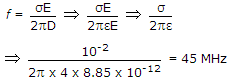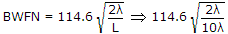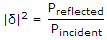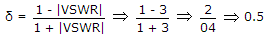# Electronics and Communication Engineering - Electromagnetic Field Theory

1.
A material has conductivity of 10-2 mho/m and a relative permittivity of 4. The frequency at which conduction current in the medium is equal to displacement current is
45 MHz
90 MHz
450 MHz
900 MHz
Explanation:

Conduction current density J = wD

J = σEσE = ωD = 2p fD2.
A transmission line is feeding 1 watt of power to a horn antenna having a gain of 10 dB. The antenna is matched to the transmission line. The total power radiated by the horn antenna into the free space is
10 watt
1 watt
0.1 watt
0.01 watt
Explanation:

Pradiated = Gain of Antenna x Input power = G.I10 log1010 . 110 watt.

3.
An end fire array consisting of several half-wave lengths of directive gain 40, then find the width of major lobe
60
50
51.25
None of the above
Explanation:.

4.
A uniform plane EM wave incident normally on a plane surface of a dielectric material is reflected with a VSWR of 3. What percentage of incident power that is reflected?
10%
25%
50%
75%
Explanation:Preflected = |δ|2 Pincident|δ|2 = 0.25 .

5.
Poynting vector is associated with which of the following?
Power flow in electromagnetic
Flux in magnetic field
Charge in electrostatic field
Current in electrostatic field